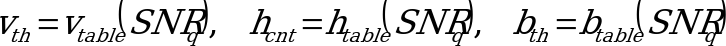06.943GPPTSVoice Activity Detector (VAD) for Adaptive Multi Rate (AMR) speech traffic channels

The quantized SNR SNRq is used to determine the respective voice metric threshold vth, hangover count hcnt, and burst count threshold bth parameters:(4.15)

where SNRQ is the index of the respective table elements. The VAD decision can then be made according to the following pseudocode:

if ( v(m) > vth + (m)) { /* if the voice metric > voice metric threshold*/
b(m) = b(m-1) + 1 /* increment burst counter */
if ( b(m) > bth ) { /* compare counter with threshold */
h(m) = hcnt /* set hangover */
}
} else {
b(m) = 0 /* clear burst counter */
h(m) = h(m-1) -1 /* decrement hangover /
if ( h(m) <= 0 ) { /* check for expired hangover /